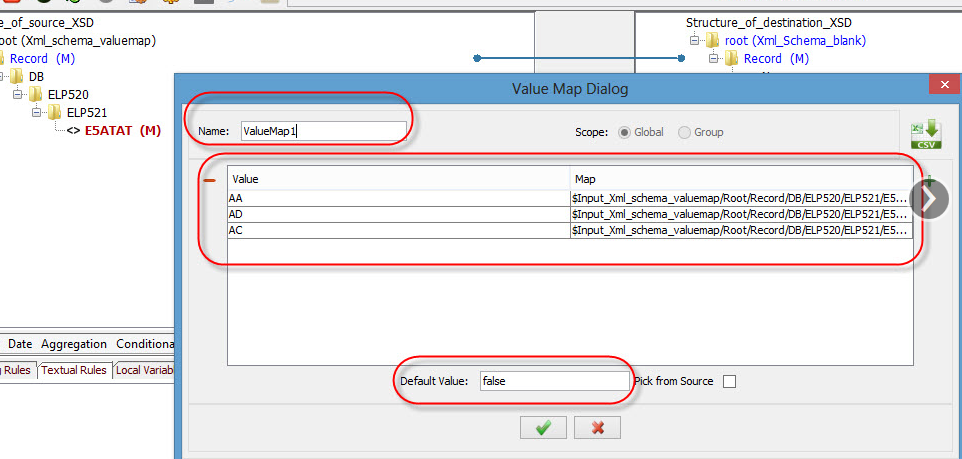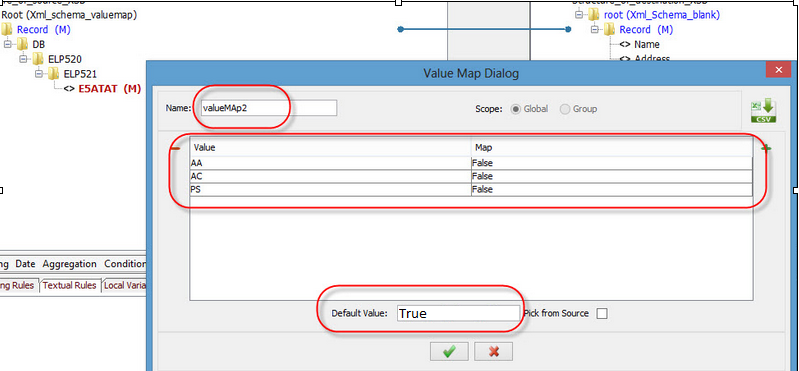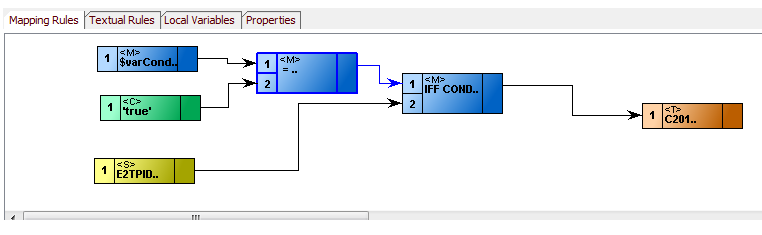# Value Map and Filter Condition

Situation 1:

I have the following IFF CONDITION which is working but I need to add a
Value Map to it to translate the value I am checking. How do I add it?

IFF CONDITION{ \$Input_XML_214_Schema_from_DB/DB/ELP520/ELP521/E5STAT =
'AA' or \$Input_XML_214_Schema_from_DB/DB/ELP520/ELP521/E5STAT = 'AD' or
\$Input_XML_214_Schema_from_DB/DB/ELP520/ELP521/E5STAT = 'AB' or
\$Input_XML_214_Schema_from_DB/DB/ELP520/ELP521/E5STAT = 'AC' or
\$Input_XML_214_Schema_from_DB/DB/ELP520/ELP521/E5STAT = 'PS' or
\$Input_XML_214_Schema_from_DB/DB/ELP520/ELP521/E5STAT = 'RB' or
\$Input_XML_214_Schema_from_DB/DB/ELP520/ELP521/E5STAT = 'X9' }
VALUE=[\$Input_XML_214_Schema_from_DB/DB/ELP520/ELP521/E5STAT ] OTHERWISE
VALUE=['false' ]

Strategy:

According to our understanding you want to map the value based on a condition through a value Map. This can be achieved by the below steps:

1. Create a local ValueMap1 in which provide the value as AA,AD,AB,AC,PS,RB,X9.

2. Now Map all the values to "\$Input_XML_214_Schema_from_DB/DB/ELP520/ELP521/E5STAT".

3. Provide the Default Value as "False".

4. Save it.

5. Then use this value map in the textual rule on the field you want to map.

For example:

ValueMap1(\$Input_XML_214_Schema_from_DB/DB/ELP520/ELP521/E5STAT)Situation 2:

The above IFF CONDITION, I need to reverse it for the AT701 to state the
same thing but instead of equal I need not not equal. I tried != but it
does not work. I do not get an error but it just does not work. How can I
do the above with not equal because there are to many to check if I try to
use the equal?

Strategy:

1. Create a local ValueMap2 in which provide the value as AA,AD,AB,AC,PS,RB,X9.

2. Now Map all the values to False

3. Provide the Default Value as "True"

4. Save it.On AT701, then duplicate on the other nodes -
1) Set valuemap2 to the global variable "varCondition"
2) Choose the boolean function Equals and set the inputs as varCondition and the constant 'true'
3) Choose the Conditional IFF Condition for filtering records
4) Set the first input as the output of the boolean function and set the second input as D1
5) Map the output of the IFF Condition to the AT701